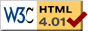### Journée-séminaire de combinatoire

#### (équipe CALIN du LIPN, université Paris-Nord, Villetaneuse)

Le 19 décembre 2017 à 14h00 en B107, Juan Vera Lizcano nous parlera de : Phase transition for the hard-core model in the 2-dimensional lattice

Résumé : The hard-core gas model is a natural combinatorial problem that has played an important role in the design of new approximate counting algorithms and for understanding computational connections to statistical physics phase transitions. For a graph G = (V, E) and a fugacity t > 0, the hard-core model is defined on the set of independent sets of G where each independent set I has weight w(I) = |I|^t . The equilibrium state of the system is described by the Gibbs distribution in which each independent set I has probability ~ w(I). A long-standing open problem to establish the critical fugacity t* for the hard-core gas model on the 2-dimensional integer lattice Z^2. In particular, t* is the conjectured critical point for the phase transition between uniqueness of infinite-volume Gibbs measures on Z^2 when t < t* and non-uniqueness when t > t*. Empirical results identified the critical point t* ~ 3.79. The best known bounds show 2.538 < t* < 5.3646. In this talk the techniques to obtain these bounds will be discussed. Special emphasis will be given to the lower bound, which is based on connections between the decay of correlations on the lattice and on its self-avoiding walk tree.

 Dernière modification : Monday 24 January 2022Contact pour cette page : Cyril.Banderier at lipn.univ-paris13.fr